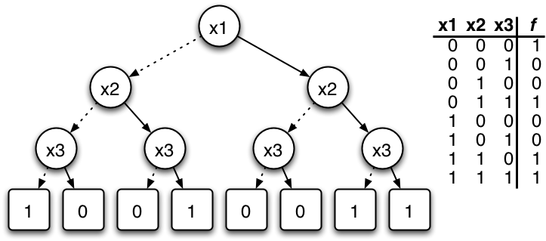# Efficient Minimization of Conflicting Assumptions in MiniSAT

Boolean Satisfiability (SAT) is the problem of deciding if there is an assignment to the variables of a Boolean formula such that the formula evaluated to TRUE. SAT is the classical NP-complete problem, and so it is unlikely that there is a polynomial-time algorithm that solves every SAT instance. Nevertheless, there are very efficient heuristic SAT-algorithms (SAT-solvers) that are able to solve practical instances with millions of variables and clauses. Today SAT-solvers are heavily used for many tasks, including hardware and software verification, artificial intelligence, planning, and so on. MiniSAT is a powerful SAT-solver available as free and open source software.

Modern SAT-based applications often involve solving formulas under "assumptions", which is a simple mechanism to restrict values of a subset of variables. When the formula under assumptions is unsatisfiable, it is often very important to know which of the assumptions are responsible for unsatisfiability, and to minimize the set of conflicting assumptions as much as possible. Specifically MiniSAT has the capability to return a subset of assumptions sufficient for unsatisfiability, however does not provide any guarantees on the minimality of this subset.

From the practical standpoint the goal of the project is to extend MiniSAT with the capability to minimize a set of conflicting assumptions, exploiting known algorithms and optimizations for a related problem of minimizing the set of clauses of an unsatisfiable CNF formula. Specifically, there is a large body of research on the latter problem, and the plan is to take various optimizations one-by-one and apply to the problem at hand.Prerequisites: Any programming language with ability to learn new concepts quickly